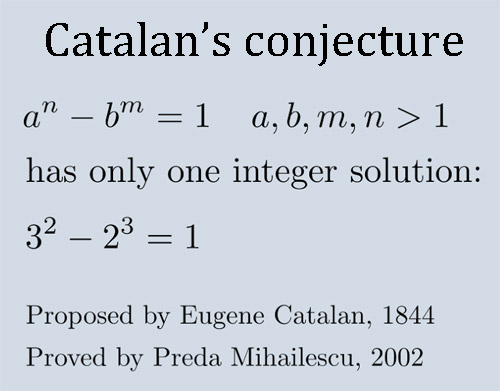A# Catalan's conjectureCatalan's conjecture is the hypothesis, put forward by the Belgian mathematician Eugène Catalan (1814–1894) in 1844, that 8 (= 23) and 9 (= 32) are the only pair of consecutive powers. In other words, the Catalan equation for prime numbers n and m and positive integers x and y:

a n - b m = 1

has only the one solution:

32 - 23 = 1.

In 1976 R. Tijdeman took the first major step toward showing this is true by proving that for any solution, y q is less than e to the power e to the power e to the power e to the power 730 (a huge number!). Since then this bound has been reduced many times, and it is now know that the larger of p and q is at most 7.78 × 1016 and the smaller is at least 107. On Apr 18, 2002, the Romanian number theorist Preda Mihailescu sent a manuscript to several mathematicians with a proof of the entire conjecture together with an analysis by Yuri Bilu. This proof was verified other mathematicians and published in 2004.

Solutions to Catalan's conjecture and Fermat's last theorem are special cases of the Fermat-Catalan equation:

x p + y q = z r

Where x, y, and z are positive, coprime integers and the exponents are all primes with

1/p + 1/q + 1/r < 1.

The Fermat-Catalan conjecture is that there are only finitely many solutions to this system. These solutions include: 1p + 23 = 32 (p ≥ 2); 25 + 72 = 34; 132 + 73 = 29; 27 + 73 = 712; 35 + 173 = 1222; 338 + 15490342 = 156133; 14143 + 22134592 = 657; 92623 + 153122832 = 1137; 177 + 762713 = 210639282; and 438 + 962223 = 300429072.

### Reference

1. Ribenboim, P. Catalan's Conjecture. Boston, Mass.: Academic Press, 1994.